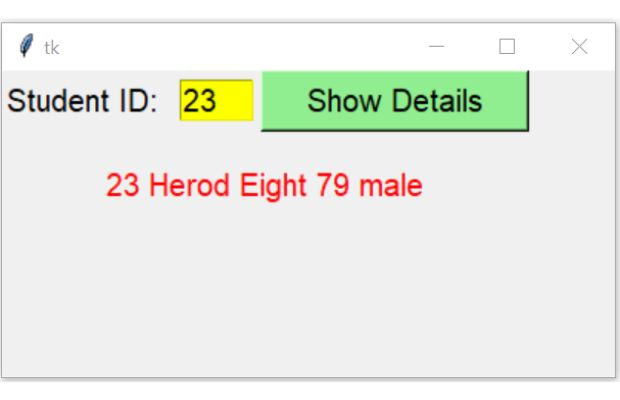# Displaying record from sqlite table based on user entered ID

Basics of Python Tkinter

Display records from sqlite Student table in Tkinter window by using entered ID of the row.## Connect to sqlite database

We connected to sqlite database by using database name.
``````import sqlite3
my_path="D:\\testing\\sqlite\\my_db.db" #Change the path
my_conn = sqlite3.connect(my_path)``````
We will use my_conn in our further script as the connection object to get our records.

Tkinter to read student id & display matching record from SQLite database table by executing query

First add t1 the Text box to receive student id from the user. This id we will use to collect matching record from the student table.
We will add one Lebel l1 to display text Enter Student ID: before the text box t1.
``````# add one Label
l1 = tk.Label(my_w,  text='Student ID: ',font=20 )

t1 = tk.Text(my_w,  height=1, width=4,bg='yellow',font=22)
We need one more Label ( l2) to display the returned details of the student. This Label initially will display the string Output.
We will be displaying data as we are getting from SQLite table, so we will declare one tkinter string variable ( StringVar() ) connected to our Label. We declared my_str = tk.StringVar() for this.
``````my_str = tk.StringVar()
l2 = tk.Label(my_w,  textvariable=my_str,font=20,fg='red' )

my_str.set("Output")``````
We will add one Button b1 to pass user entered data to a function to get the record details.

## click event of the button b1

Once the Button is clicked it will read the data entered in the text box t1 and pass it to the user defined function my_details().
``````b1 = tk.Button(my_w, text='Show Details', width=15,bg='lightgreen',font=22,
command=lambda: my_details(t1.get('1.0',END)))
b1.grid(row=1,column=3)``````

## my_details()

On click of the Button b1 , my_details() function will receive the string id as input parameter. This is the id of the student for which all the detail of the record will be taken from student table and displayed by using l2 label.

Here data ( id ) is entered by user through the text field ( t1 ). So before using this data in our database Query, we must check this input data.
Inside the function my_details() we will use try except code blocks to check if the user entered data id is Integer or not. If it is not integer then except: part of the code block will display message Check input.
`````` try:
val = int(id) # check input is integer or not
except:
my_str.set("Check input")``````
Inside the try block ( the input id is integer ) we will keep one more try and except. In this try block we will keep the database handling part and in except block we will display message Database error. The full code of the function my_details() is here.
``````def my_details(id):
try:
val = int(id) # check input is integer or not
try:
my_data=(val,)
q="SELECT * FROM student WHERE id= ?"
my_cursor=my_conn.execute(q,my_data)
data_row=my_cursor.fetchone()
my_str.set(data_row)

except sqlite3.Error as my_error:
print("error: ",my_error)
except:
my_str.set("Check input")``````
We used SQL with WHERE to collect details of the record by using student id from the student table.

Note that SQLite database executes the query part only and return us one result set. This result set contains details of our row data of input ID.
We used SQLite result set i.e my_cursor here.
The full code is here
``````import sqlite3
my_path="D:\\testing\\sqlite\\my_db.db" #Change the path
my_conn = sqlite3.connect(my_path)
###### end of connection ####

##### tkinter window ######
import tkinter  as tk
from tkinter import *
####### end of connection ####

my_w = tk.Tk()
my_w.geometry("400x200")

l1 = tk.Label(my_w,  text='Student ID: ',font=20 )

t1 = tk.Text(my_w,  height=1, width=4,bg='yellow',font=22)

b1 = tk.Button(my_w, text='Show Details', width=15,bg='lightgreen',font=22,
command=lambda: my_details(t1.get('1.0',END)))
b1.grid(row=1,column=3)

my_str = tk.StringVar()
l2 = tk.Label(my_w,  textvariable=my_str,font=20,fg='red' )

my_str.set("Output")

def my_details(id):
try:
val = int(id) # check input is integer or not
try:
my_data=(val,)
q="SELECT * FROM student WHERE id= ?"
my_cursor=my_conn.execute(q,my_data)
data_row=my_cursor.fetchone()
my_str.set(data_row)

except sqlite3.Error as my_error:
print("error: ",my_error)
except:
my_str.set("Check input")
my_w.mainloop()``````

## Using SQLAlchemy

Here the connection string ( to generate my_conn ) and the error handling within try except code block will only change. Other parts remain same.
Full code is here
``````from sqlalchemy import create_engine
from sqlalchemy.exc import SQLAlchemyError
my_path="D:\\testing\\sqlite\\my_db.db" #Change the path
my_conn = create_engine("sqlite:///" + my_path)
###### end of connection ####

##### tkinter window ######
import tkinter  as tk
from tkinter import *
####### end of connection ####

my_w = tk.Tk()
my_w.geometry("400x200")

l1 = tk.Label(my_w,  text='Student ID: ',font=20 )

t1 = tk.Text(my_w,  height=1, width=4,bg='yellow',font=22)

b1 = tk.Button(my_w, text='Show Details', width=15,bg='lightgreen',font=22,
command=lambda: my_details(t1.get('1.0',END)))
b1.grid(row=1,column=3)

my_str = tk.StringVar()
l2 = tk.Label(my_w,  textvariable=my_str,font=20,fg='red' )

my_str.set("Output")

def my_details(id):
try:
val = int(id) # check input is integer or not
try:
my_data=(val,)
q="SELECT * FROM student2 WHERE id= ?"
my_cursor=my_conn.execute(q,my_data)
data_row=my_cursor.fetchone()
my_str.set(data_row)

except SQLAlchemyError as e:
error=str(e.__dict__['orig'])
print(error)
except:
my_str.set("Check input")
my_w.mainloop()``````

Subscribe to our YouTube Channel here

## Subscribe

* indicates required
Subscribe to plus2netplus2net.com